Contents Previous Next

## 8.4 Scatter plots

Scatter plots are very simple; they plot a number of points specified by their X- and Y-coordinate. Each point is stroked on the image with a mark as with line plots. The stroked marks can also be connected with an optional line.

Note: Even though it is only scatter plot that was designed to be used with X,Y plots it is perfectly possible to use use both X,Y coordinates for bar and line plots as well.

Even though you would normally supply X-coordinates it is still perfectly possible to use a text-scale for X-coordinates to just enumerate the points. This is especially useful when using the "Impulse" type of scatter plot as is shown below.

Scatter pots are created by including the jpgraph extension "jpgraph_scatter.php" and then creating an instance of plot type of ScatterPlot(). To specify the mark you want to use you access the mark with the instance variable "mark" in the scatter plot. The default is to use an unfilled small circle.

To create a scatter plot you will create an instance

A simple example using just default values will illustrate this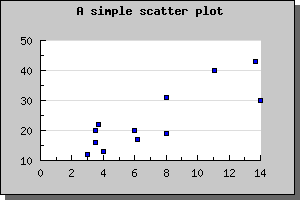Figure 57: The simplest possible scatter plot [src]

We can easily adjust the size and colors for the markers to get another effect as shown below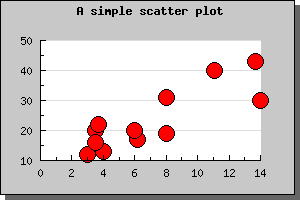Figure 58: The simplest possible scatter plot with adjusted marks [src]

Another possible variant of scatter plot is impulse-scatter plots. This is a variant of normal scatter plot where each mark have a line from the mark to the Y=0 base line. To change a scatter plot into an impulse scatter plot you have to call the method SetImpuls() on the scatter plot.

This type of plots are often used to illustrate signals in conjunction with digital signal processing. The following two examples illustrates simple use of impulse plots.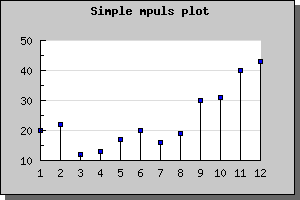Figure 59: A simple impuls plot [src]

The next example shows how to modify the color and width of the impulse plot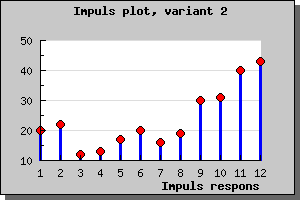Figure 60: A modified impuls plot [src]

Note: You may draw impulse graphs without any mark by specifying the mark type as (-1) . That way only the impulse lines will be drawn.

As a final touch we show two more advanced impulse graphs . In these graphs we have used more advanced formatting for the Y-axis labels as well as adjusted the position of the axis position.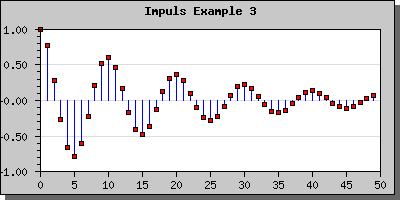Figure 61: In this imuplsplot we have adjusted the position of the X-axis to the bottom and also added more decimals to the labels on the Y-axis [src]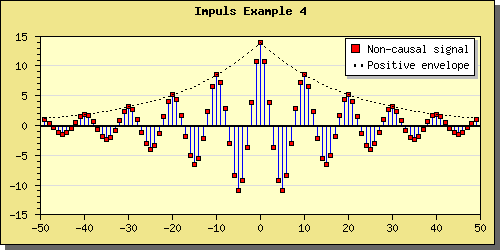Figure 62: In this impuls plot we have also added a lineplot with a dotted line style. [src]

Contents Previous Next